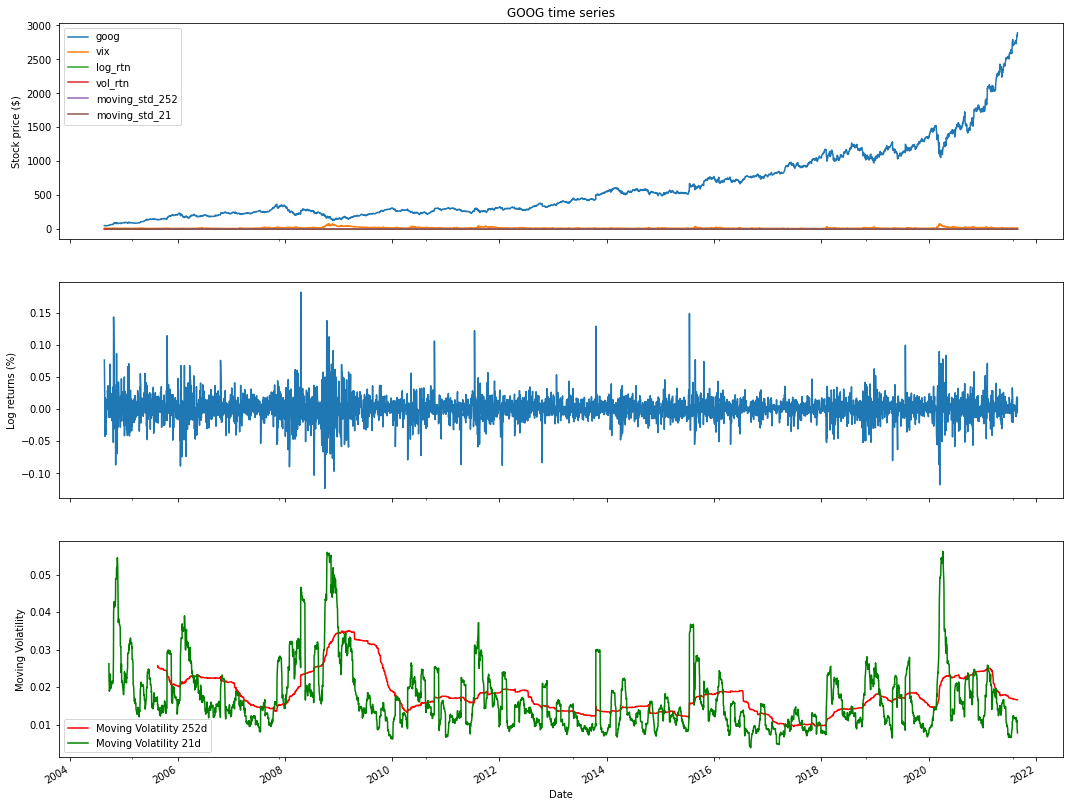# Google stockprice analysis in python

## What are alternative sources to get financial data?

### Google is an American multinational company specializing in Internet services.

Analyzing Google's stock price using Python involves collecting historical price data, performing data analysis, and creating visualizations to gain insights into the company's stock performance. Here's a step-by-step guide on how to conduct Google stock price analysis in Python:

Import Libraries:
Start by importing the necessary Python libraries for data manipulation, analysis, and visualization. Commonly used libraries include pandas, numpy, matplotlib, and yfinance to fetch historical data:

python
Copy code
import pandas as pd
import numpy as np
import matplotlib.pyplot as plt
import yfinance as yf
Data Retrieval:
Use the yfinance library or other financial data sources to fetch historical data for Google's stock. Specify the start and end dates for the data you want to analyze:

python
Copy code
Data Exploration:
Explore the fetched data to understand its structure and contents. Use functions like head(), tail(), describe(), and info() to inspect the dataset:

python
Copy code
Data Visualization:
Create visualizations to analyze the historical performance of Google's stock. Common visualizations include line charts to visualize price movements:

python
Copy code
plt.figure(figsize=(12, 6))
plt.xlabel('Date')
plt.ylabel('Price')
plt.legend()
plt.show()
Technical Analysis (Optional):
Perform technical analysis by calculating and visualizing technical indicators like moving averages, relative strength index (RSI), and MACD. Libraries like ta-lib can be used for these calculations.

Statistical Analysis (Optional):
Conduct statistical analysis to calculate summary statistics, volatility measures, and correlations with other assets. numpy and pandas are useful for these calculations.

Sentiment Analysis (Optional):
Consider incorporating sentiment analysis of news articles or social media data related to Google to understand market sentiment's impact on the stock price.

Fundamental Analysis (Optional):
Analyze fundamental factors affecting Google, such as earnings reports, revenue growth, and market share, which can influence the stock's performance.

Prediction and Forecasting (Optional):
You can use time series forecasting techniques like ARIMA or machine learning models to make predictions about future Google stock price movements.

Risk Management and Decision Making:
Based on your analysis, formulate investment strategies, set risk management parameters, and make informed investment decisions regarding Google's stock.

Keep your analysis up to date with the latest data to adapt to changing market conditions and make timely decisions.

Remember that investing in stocks carries risks, and it's crucial to do thorough research, consider factors like company news and market trends, and potentially consult with financial experts before making investment decisions based on your analysis of Google's stock price or any other stock.
What are alternative sources to get financial data?
There are a number of alternative sources like Quandal,intrinio,google others.

### How to get financial data from Google in Python?

!pip install yfinance
import pandas as pd
import numpy as np
import yfinance as yf
start='2020-01-01',
end='2021-08-28',
progress=False)
df.tail(9)

Date
2021-08-172763.8200682774.3701172735.7500002746.0100102746.0100101063600
2021-08-182742.3100592765.8798832728.4199222731.3999022731.399902746700
2021-08-192709.3500982749.0400392707.1201172738.2700202738.270020914800
2021-08-202741.6599122772.2900392729.3400882768.7399902768.739990778200
2021-08-232779.9699712843.5400392774.9589842821.9899902821.9899901054500
2021-08-242830.8701172860.1499022827.0700682847.9699712847.969971756300
2021-08-252857.6599122866.2600102848.7900392859.0000002859.000000641900
2021-08-262852.3701172862.6960452841.8300782842.4599612842.45996174610
import matplotlib.pyplot as plt

### How to plot google close price?

df['Close'].plot(figsize=(12,8))
<matplotlib.axes._subplots.AxesSubplot at 0x7fb1102efb90>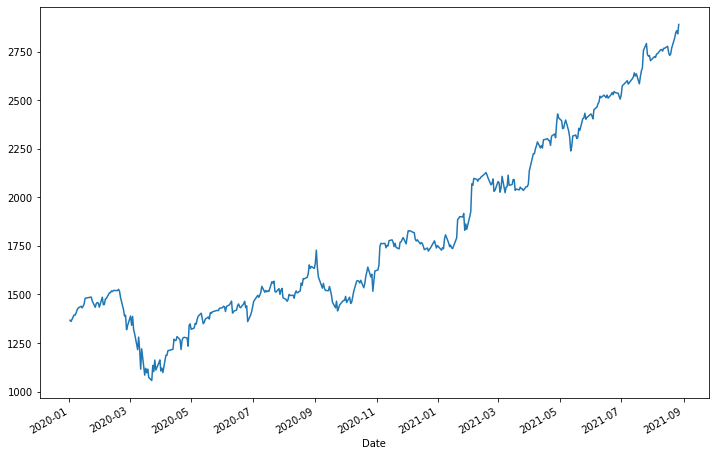### How to plot Google close price and volume?

df[['Close''Volume']].plot(subplots=True, style='b',
figsize=(128))
array([<matplotlib.axes._subplots.AxesSubplot object at 0x7fb1101eed90>,
<matplotlib.axes._subplots.AxesSubplot object at 0x7fb10fd55d90>],
dtype=object)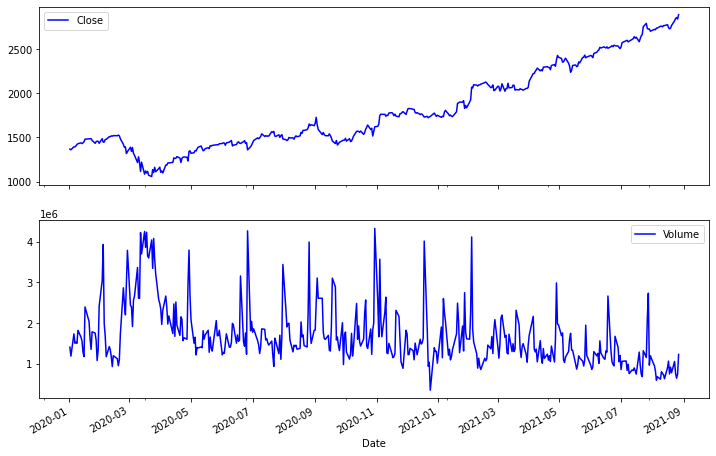### How to describe the mean median and other statistics?

df.describe()
df['simple_rtn'] = df.Close.pct_change()
df['log_rtn'] = np.log(df.Close/df.Close.shift(1))
df['log_rtn'].plot(subplots=True, style='b',
figsize=(128))
array([<matplotlib.axes._subplots.AxesSubplot object at 0x7fb10fc48150>],
dtype=object)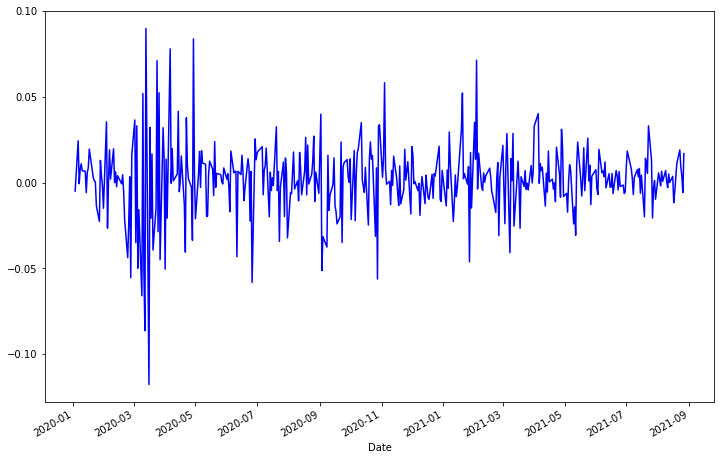### How to convert Stock prices into log and simple returns in Python?

df['log_rtn'].tail(12)
Date 2021-08-12 0.005071 2021-08-13 0.000119 2021-08-16 0.003678 2021-08-17 -0.011698 2021-08-18 -0.005335 2021-08-19 0.002512 2021-08-20 0.011066 2021-08-23 0.019050 2021-08-24 0.009164 2021-08-25 0.003865 2021-08-26 -0.005802 2021-08-27 0.016936 Name: log_rtn, dtype: float64
from plotly.offline import iplot, init_notebook_mode
init_notebook_mode()
df_rolling = df[['simple_rtn']].rolling(window=21) \
.agg(['mean''std'])
df_rolling.columns = df_rolling.columns.droplevel()
df_outliers = df.join(df_rolling)
import pandas as pd
import numpy as np
import yfinance as yf
import seaborn as sns
import scipy.stats as scs
import statsmodels.api as sm
import statsmodels.tsa.api as smt
start='1985-01-01',
end='2021-08-28',
progress=False)
df.columns = df.columns.droplevel(0)
df = df.rename(columns={'GOOG''goog''^VIX''vix'})
df.tail()
df['log_rtn'] = np.log(df.goog / df.goog.shift(1))
df['vol_rtn'] = np.log(df.vix / df.vix.shift(1))
df.dropna(how='any', axis=0, inplace=True)
corr_coeff = df.log_rtn.corr(df.vol_rtn)
corr_coeff = df.log_rtn.corr(df.vol_rtn)
ax = sns.regplot(x='log_rtn', y='vol_rtn', data=df,
line_kws={'color''red'})
ax.set(title=f'GOOG vs. VIX ($\\rho$ = {corr_coeff:.2f})',
ylabel='VIX log returns',
xlabel='GOOG log returns')
[Text(0, 0.5, 'VIX log returns'),
Text(0.5, 0, 'GOOG log returns'),
Text(0.5, 1.0, 'GOOG vs. VIX ($\\rho$ = -0.48)')]

### How to compare the stock price of Google and vix?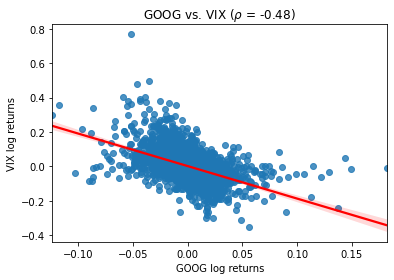r_range = np.linspace(min(df.log_rtn), max(df.log_rtn), num=1000)
mu = df.log_rtn.mean()
sigma = df.log_rtn.std()
norm_pdf = scs.norm.pdf(r_range, loc=mu, scale=sigma)
fig, ax = plt.subplots(12, figsize=(168))
# histogram
sns.distplot(df.log_rtn, kde=False, norm_hist=True, ax=ax)
ax.set_title('Distribution of GOOG returns', fontsize=16)
ax.plot(r_range, norm_pdf, 'g', lw=2,
label=f'N({mu:.2f}{sigma**2:.4f})')
ax.legend(loc='upper left');
# Q-Q plot
qq = sm.qqplot(df.log_rtn.values, line='s', ax=ax)
ax.set_title('Q-Q plot', fontsize = 16)
distplot is a deprecated function and will be removed in a future version. Please adapt your code to use either displot (a figure-level function with similar flexibility) or histplot (an axes-level function for histograms).
How to plot google distribution of stock price and Q-Q plot?
Text(0.5, 1.0, 'Q-Q plot')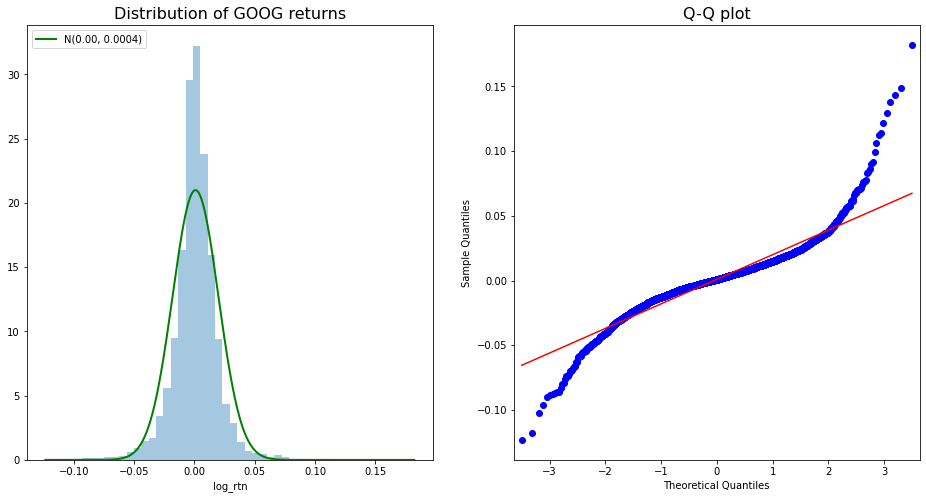### How to plot Google's daily stock return?

df.log_rtn.plot(title='Daily GOOG returns')
<matplotlib.axes._subplots.AxesSubplot at 0x7fb0ecf04dd0>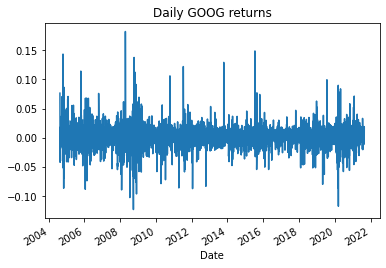N_LAGS = 50
SIGNIFICANCE_LEVEL = 0.05
acf = smt.graphics.plot_acf(df.log_rtn,
lags=N_LAGS,
alpha=SIGNIFICANCE_LEVEL
)

### How to plot Google's absolute return and autocorrelation?

fig, ax = plt.subplots(21, figsize=(1210))
smt.graphics.plot_acf(df.log_rtn ** 2, lags=N_LAGS,
alpha=SIGNIFICANCE_LEVEL, ax = ax)
ax.set(title='Autocorrelation Plots',
ylabel='Squared Returns')
smt.graphics.plot_acf(np.abs(df.log_rtn), lags=N_LAGS,
alpha=SIGNIFICANCE_LEVEL, ax = ax)
ax.set(ylabel='Absolute Returns',
xlabel='Lag')
[Text(0, 0.5, 'Absolute Returns'), Text(0.5, 0, 'Lag')]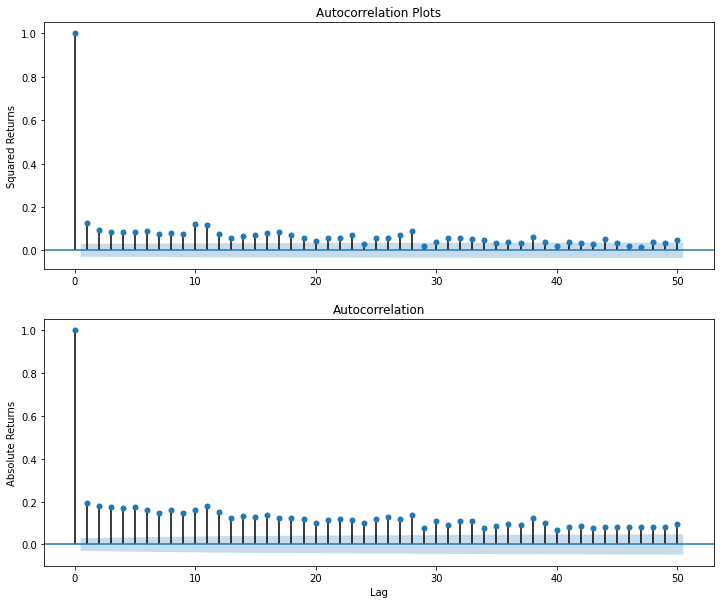### How to plot Google 252 days and 21 days moving volatility?

df['moving_std_252'] = df[['log_rtn']].rolling(window=252).std()
df['moving_std_21'] = df[['log_rtn']].rolling(window=21).std()
fig, ax = plt.subplots(31, figsize=(1815),
sharex=True)
df.plot(ax=ax)
ax.set(title='GOOG time series',
ylabel='Stock price (\$)')
df.log_rtn.plot(ax=ax)
ax.set(ylabel='Log returns (%)')
df.moving_std_252.plot(ax=ax, color='r',
label='Moving Volatility 252d')
df.moving_std_21.plot(ax=ax, color='g',
label='Moving Volatility 21d')
ax.set(ylabel='Moving Volatility',
xlabel='Date')
ax.legend()
<matplotlib.legend.Legend at 0x7fb0e4997e50>`